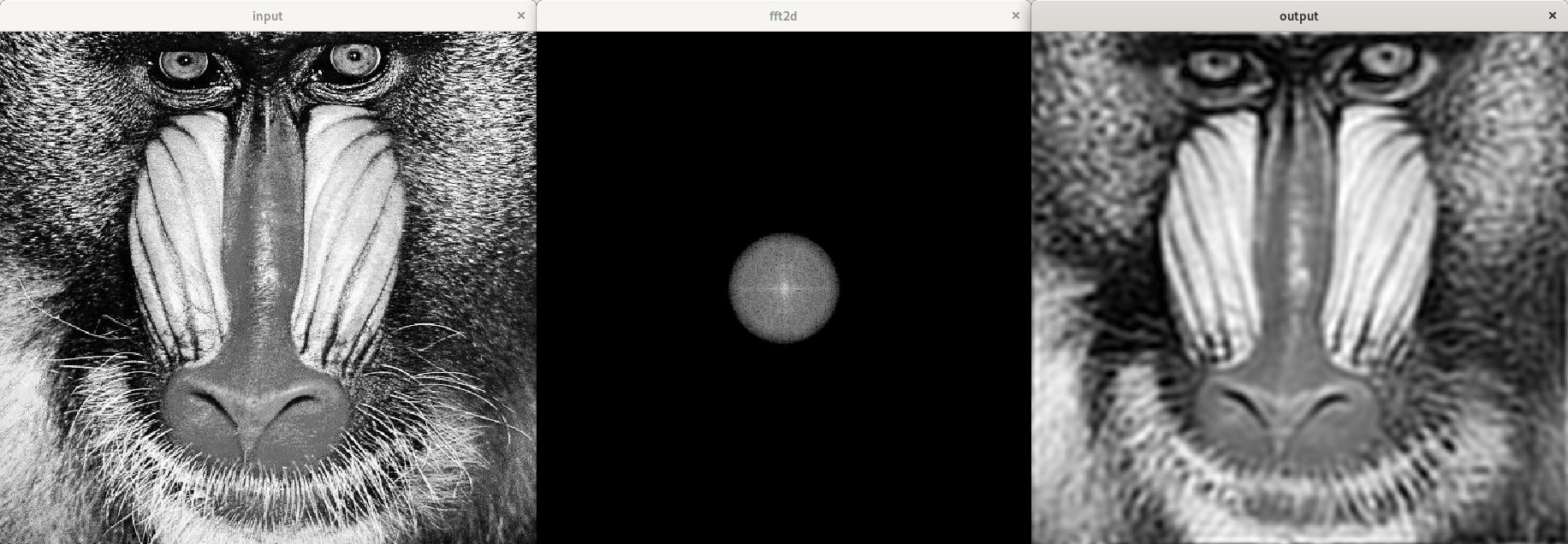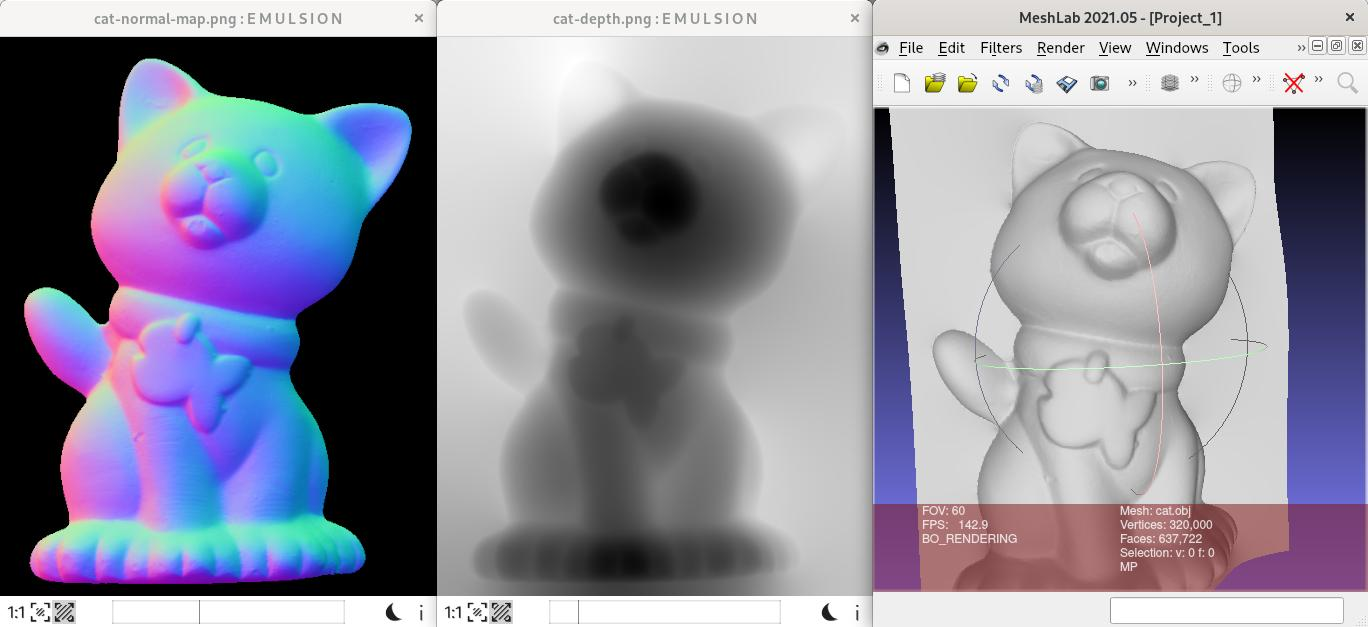# 2D Fourier transform for images

Matthieu Pizenberg
Easy to use 2D spectral transforms for images, such as Fourier (FFT) and cosine (DCT) transforms
2021

This crate aims to provide easy to use 2D spectral transforms for images, such as Fourier (FFT) and cosine (DCT) transforms. By default, only the Fourier transforms are availables. The cosine transform is available with the `rustdct` feature, named after the corresponding optional dependency.

By default, only implementations on mutable slices `&mut [f64]` are available, inside the `fft2d::slice` module of this crate. Implementations of the transforms are also available for nalgebra matrices in the `fft2d::nalgebra` module, if the `nalgebra` feature is activated.

Here is a partial extract of code for the `low_pass` filtering example with the input and output images, just to get a sense of the API.``````// Compute the 2D fft of the complex image data (beware of the transposition).
fft_2d(width, height, &mut img_buffer);

// Shift opposite quadrants of the fft (like matlab fftshift).
img_buffer = fftshift(height, width, &img_buffer);

// Apply a low-pass filter.
let low_pass = low_pass_filter(height, width);
let fft_low_pass: Vec<Complex<f64>> =
low_pass.iter().zip(&img_buffer)
.map(|(l, b)| l * b).collect();

// Invert the FFT back to the spatial domain of the image.
img_buffer = ifftshift(height, width, &fft_low_pass);
ifft_2d(height, width, &mut img_buffer);

// Normalize the data after FFT and IFFT.
let fft_coef = 1.0 / (width * height) as f64;
for x in img_buffer.iter_mut() { *x *= fft_coef; }
``````

## Examples

There are a few examples showing how to use the API, located in the `examples/` folder.

### Low pass filter via Fourier transform

This example shows how to apply a low pass filter on images. It is present both for the mutable slice API at `examples/low_pass.rs` and for the nalgebra API at `examples/low_pass_nalgebra.rs`. Example results are visible in the figure above in the readme. You can compile and run the example with the following command:

``````cargo run --release --example low_pass
``````

### Image resizing via 2D cosine transform

This example shows how to use a 2D cosine transform to resize an image. It consists in performing a DCT, then truncating (or expanding) the image buffer, and performing and inverse DCT. You can compile and run the example with the following command:

``````cargo run --release --features rustdct --example dct_resize
``````

### Normal integration to generate a depth map (or a 3D mesh)

In this example, we start from a normal map, which is an image encoding the (x,y,z) components of a surface normals into the RGB components of an image, and we integrate that normal map to get a depth map containing an estimated depth Z at each pixel. One algorithm to integrate those normals consists in writing a Poisson solver (as in Poisson equation) based on a 2D DCT. The relevant code is located in the `dct_poisson` function of the `examples/normal_integration.rs` file. You can compile and run the example with the following command:

``````cargo run --release --features rustdct,nalgebra --example normal_integration
``````The cat normal map used in this example comes from Harvard's photometric stereo dataset.

The code for the normal integration is a Rust implementation of the method presented in the following paper. Big thanks to Yvain Quéau (@yqueau) for the help in setting up this port of his matlab code.

Normal Integration: a Survey - Queau et al., 2017